How to Learn in 24 Hours?The Rapid Learning Movie

 Need Help? M-F: 9am-5pm(PST): Toll-Free: (877) RAPID-10 US Direct: (714) 692-2900 Int'l: 001-714-692-2900 24/7 Online Technical Support: The Rapid Support Center Secure Online Order:Need Proof? Testimonials by Our Users

 Rapid Learning Courses: MCAT in 24 Hours (2015-16) USMLE in 24 Hours (Boards) Chemistry in 24 Hours Biology in 24 Hours Physics in 24 Hours Mathematics in 24 Hours Psychology in 24 Hours SAT in 24 Hours ACT in 24 Hours AP in 24 Hours CLEP in 24 Hours DAT in 24 Hours (Dental) OAT in 24 Hours (Optometry) PCAT in 24 Hours (Pharmacy) Nursing Entrance Exams Certification in 24 Hours eBook - Survival Kits Audiobooks (MP3)

 Tell-A-Friend: Have friends taking science and math courses too? Tell them about our rapid learning system.Home »  Mathematics »  Introductory Statistics

Regression Inference

Topic Review on "Title":

Some Definitions

• Regression: X, the independent variable, the explanatory variable or the predictor variable. Y, the dependent variable, the response variable or the predicted variable. Given X, we will be able to predict Y.
• Deterministic Model: y = a + bx
• Probabilistic Model: y = a + bx+ e , where e ~N(0,s2)

Least Square Estimators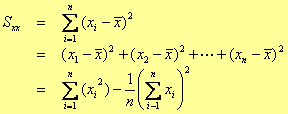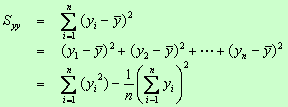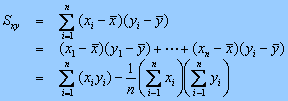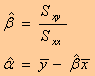ANOVA

• SST=the total variation in the experiment.
• SST=SSR+SSE
• SSR (sum of squares for regression): measures the variation explained by regression model.
• SSE (sum of squares for error): measures the variation not explained by x.

ANOVA Table

 Source df SS MS Test Statistics (Mean Squares) F Regression 1 SSR SSR/(1) MSR/MSE Model (=MSR) Error n - 2 SSE SSE/(n-2) (=MSE) Total n -1 SST

F Test

• F test shall be used to test whether the regression model fit well or not. If the model fit well, the test statistics F will be large. (This test is equivalent to t-test for t2 = F)
• H0: The regression model fits well.
• Ha: The regression model does not fit well.
• F=MSR/MSE
• Reject H0 if F>Fa,1,n-2
• a is the probability of a type I error

T test and Confidence level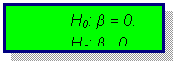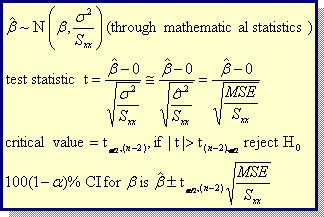Rapid Study Kit for "Title":
 Flash Movie Flash Game Flash Card Core Concept Tutorial Problem Solving Drill Review Cheat Sheet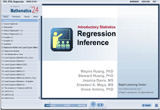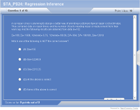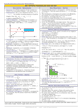"Title" Tutorial Summary : This tutorial specifically describes the regression inference. In statistics, we frequently measure two or more variables on the same experimental unit. We do this to explore the nature of the relationship among these variables.  Regression inference is to use knowledge of independent variable(s) to predict dependent variable. By completing this course, you will learn about the regression inference including regression models and least square method, the analysis of variance (ANOVA), testing regression model, assumptions and estimation and prediction

 Tutorial Features: Specific Tutorial Features: Animated examples showing the operation of least square method is presented in the tutorial. Step by step analysis of ANOVA is presented and served as a base for the subsequent hypothesis testing for the regression model. Series Features: Concept map showing inter-connections of new concepts in this tutorial and those previously introduced. Definition slides introduce terms as they are needed. Visual representation of concepts Animated examples—worked out step by step A concise summary is given at the conclusion of the tutorial.

 "Title" Topic List: Regression Models and Least Square Method The Analysis of Variance (ANOVA) Testing Regression Model (F test, t test and confidence interval) Assumptions Estimation and Prediction

See all 24 lessons in Introductory Statistics, including concept tutorials, problem drills and cheat sheets:
Teach Yourself Introductory Statistics Visually in 24 Hours